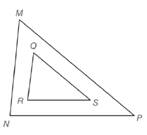Chapter 5.2, Problem 9EElementary Geometry For College St...

7th Edition
Alexander + 2 others
ISBN: 9781337614085

Solutions

Chapter
SectionElementary Geometry For College St...

7th Edition
Alexander + 2 others
ISBN: 9781337614085
Textbook Problem

Given: △ M N P ∼ △ Q R S , m ∠ M = 56 ∘ , m ∠ R = 82 ∘ , M N = 9 , Q R = 6 , R S = 7 , M P = 12 Find: a) m ∠ N c) N P b) m ∠ P d) Q STo determine

a)

To find:

mN.

Explanation

Definition used:

Similarity between Two polygons:

Two polygons are similar if and only if when all pairs of corresponding angles are congruent and all pairs of corresponding sides are proportional.

Given:

ΔMNPΔQRS,mM=56,mR=82,MN=9,QR=6,RS=7,MP=12

Calculation:

We have ΔMNPΔQRS

To determine

b)

To find:

mP.

To determine

c)

To find:

NP.

To determine

d)

To find:

QS.

Still sussing out bartleby?

Check out a sample textbook solution.

See a sample solution

The Solution to Your Study Problems

Bartleby provides explanations to thousands of textbook problems written by our experts, many with advanced degrees!

Get Started

Convert the expressions in Exercises 6584 to power form. 3x1.2213x2.1

Finite Mathematics and Applied Calculus (MindTap Course List)

Prove that cosine is a continuous function.

Single Variable Calculus: Early Transcendentals

The acceleration for a particle whose position at time t is is:

Study Guide for Stewart's Multivariable Calculus, 8th